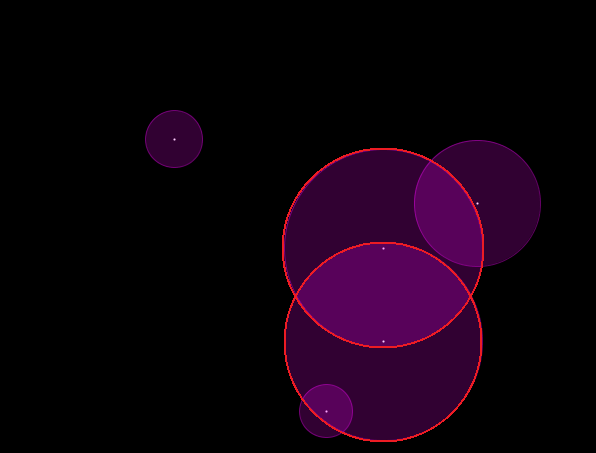#### Howdy, Stranger!

We are about to switch to a new forum software. Until then we have removed the registration on this forum.

# How to connect two or more things in one array

edited July 2018

Hello, Im trying to change colors of two (or more) cirlces when their centers are in range of each others radiuses. Something like that:I tried double for loop, but I failed.

``````Postac[] p;

void setup() {
size(600, 600);
p = new Postac;

for (int i = 0; i < p.length; i++) {
p[i] = new Postac();
}
}

void draw() {
background(0);

for (int j, i = 0; i < p.length; i++) {
p[i].display();
p[i].mouseOver();
}
}

class Postac {

int x = int(random(50, width-50)), y = int(random(50, height-50)),
radius = int(random(50, 200));
int r = 250, g = 3, b = 255;
boolean over;

void display() {
strokeWeight(2);
stroke(255);
point(x, y);

strokeWeight(1);
stroke(r, g, b, 100);
fill(r, g, b, 50);
ellipse(x, y, radius, radius);
}

void mouseOver() {

if (mouseX > x-3 && mouseX < x+3 && mouseY > y-3 && mouseY < y+3) {
r = 255;
g = 255;
b = 255;
over = true;
} else {
r = 250;
g = 3;
b = 255;
over = false;
}
}
}
``````
Tagged:

## Answers

• You store your circles in an array. You are not comparing the circles against each other. So you have:

``````  for (int j, i = 0; i < p.length; i++) {
p[i].display();
p[i].mouseOver();
}
``````

You need instead

``````void draw() {
background(0);

if(p.length>1){  //Need to have more than 1 to check overlap
for (int i = 0; i < p.length; i++) {
for (int other = i; other < p.length; other++) {
boolean res=p[i].overlapOtherCircle(p[other]);

if(res==true){
//Overlap happened: Change the color of both the 'i' and 'other' object
}
else{
//Update the field of only i object with a non-overlapping color
}
}
}
}

for (int i = 0; i < p.length; i++) {
p[i].display();
}
}
``````

and inside your class you need to define:

``````boolean overlapOtherCircle(Postac  otherObj){
return dist(x,y,otherObj.x,otherObj.y)  >  (radius/2.0 + otherObj.radius/2.0);
}
``````

Check this

www.jeffreythompson.org/collision-detection/index.php
https://forum.processing.org/two/discussion/24966/bouncing-balls-collision#latest

Notice there is a new forum at https://discourse.processing.org

Kf

• for (int other = i

for (int other = i+1

And adjust line 5

• kfrajer, it doesnt work :/

• edited July 2018

i have made couple of changes in your code and i ended up with something like this, works perfect :D

``````Postac[] p;
boolean pio,oip;             //pio - point in object  /  oip - object in point

void setup() {
size(600, 600);
p = new Postac;

for (int i = 0; i < p.length; i++) {
p[i] = new Postac();
}
}

void draw() {
background(0);

for (int i = 0; i < p.length; i++) {
for (int other = 0; other < p.length; other++) {
float d =dist(p[i].x, p[i].y, p[other].x, p[other].y);
if (d >  (p[i].radius/2))     pio = false;
if (d <= (p[i].radius/2))     pio = true;
if (d >  (p[other].radius/2)) oip = false;
if (d <= (p[other].radius/2)) oip = true;

if (p[i].over && i!=other) {
println("p[i]=", i, " ", "p[other]=", other, " ", "dist=", d, " ", "radius=", p[i].radius);

if (pio && oip) {
p[other].r = 0;
p[other].g = 255;
p[other].b = 0;
}
}
}
}
for (int i = 0; i < p.length; i++) {
p[i].display();
p[i].mouseOver();
}
}

class Postac {

int x = int(random(50, width-50)), y = int(random(50, height-50)),
radius = int(random(50, 200));
int r = 250, g = 3, b = 255;
boolean over;

void display() {
strokeWeight(2);
stroke(255);
point(x, y);

strokeWeight(1);
stroke(r, g, b, 100);
fill(r, g, b, 50);
ellipse(x, y, radius, radius);
}

void mouseOver() {

if (mouseX > x-3 && mouseX < x+3 && mouseY > y-3 && mouseY < y+3) {
r = 255;
g = 255;
b = 255;
over = true;
} else {
r = 250;
g = 3;
b = 255;
over = false;
}
}
}
``````
• Instead of

for (int other = 0;

Better

for (int other = i+1

And adjust previous for accordingly

• thanks a lot guys ;)

• I think you need a pre-loop to set all the circles to non-overlapping and then a nested loop to set the overlapping ones to the overlapping colour, you can't just have an else statement inside the nested loop.

Why not? Say you have three circles. The first two overlap, so you set them as overlapping. You then check them both against circle 3 and neither overlap with circle 3 so you set them both as non-overlapping...

Sign In or Register to comment.